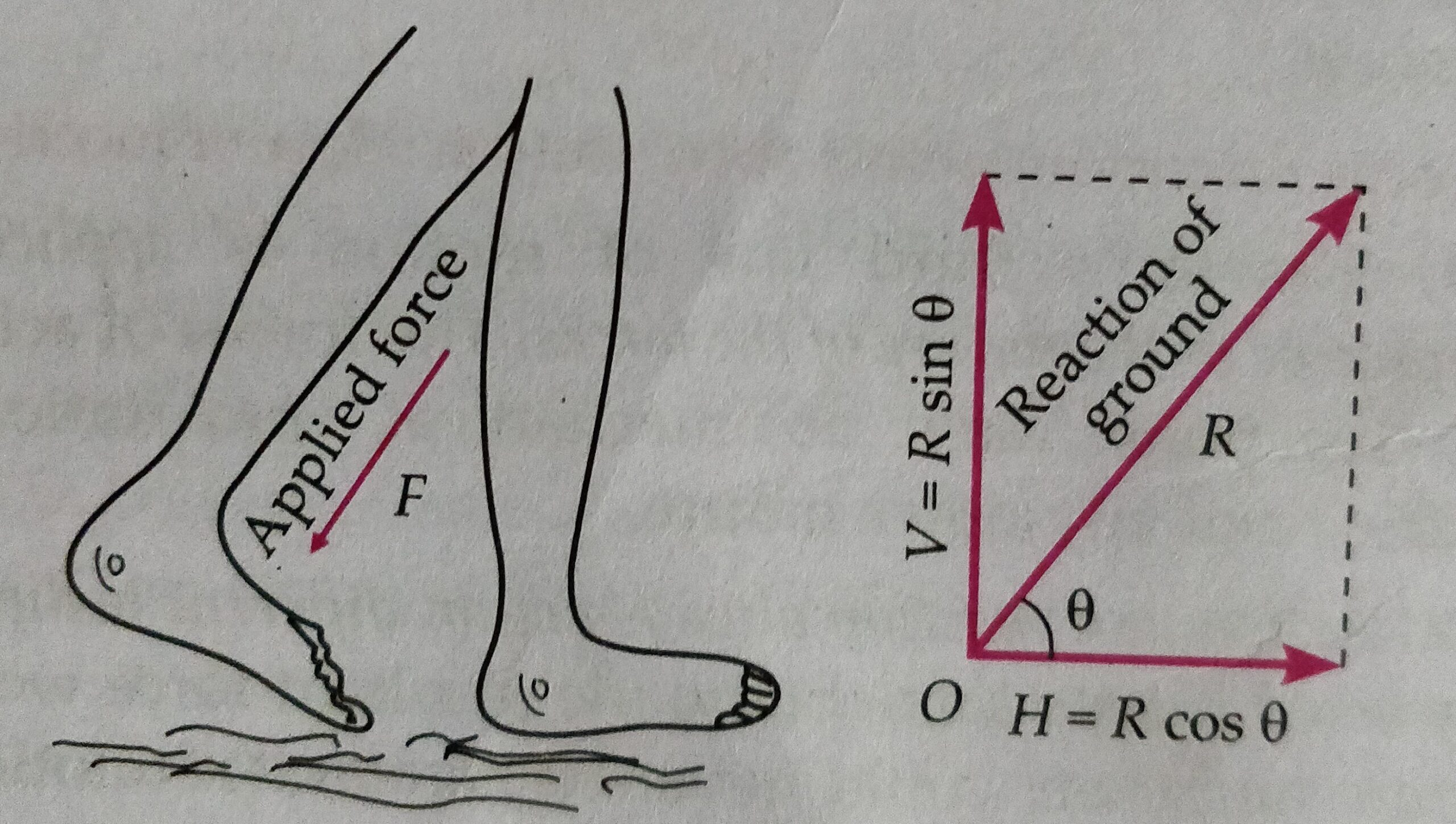# Newton’s third law of motion

In this topic we will discuss about Newton’s third law of motion and some important illustrations of Newton’s third law of motion .

Before to go with this topic students must know Newton’s first and second law .

Newton’s third law of motion-

According to this law every action has equal and opposite reaction.

Forces in nature always occur between pairs of bodies. Let two objects A and B colliding as shown in figure.FAB force on A due to B ,and FBA force on B due to A .

Then according to Newton’s third law of motion FAB = -FBA .

For example- When a man walk on the ground then man push the road backward and road push the man in forward direction. This example shows , single force never exist and force always exist in pair.

Important point of Newton’s third law we must know

(i) Action and reaction always acts on different bodies.

(ii) No action occur without reaction.

(iii) Action and reaction can’t cancel each other.

(iv) action and reaction may be mechanical, gravitational, electrical or of any other nature.

Some important illustration of Newton’s third law of motion.-

1. Object kept on the table – suppose an object of mass ‘m’ placed on the table , then the weight ‘W’ applying a force equal ti its weight W = mg,  on the surface of table , and table applying a reaction R on the book , In this condition W = -R.1. 2. Walking of a man on the ground –

While walking we press the ground F ( action ) with our feet in the slightly backward direction and ground  exert an equal reaction R , as shown in figure . then R has two component H = R cosϴ in horizontal direction  and V= R sinϴ in vertical direction . V balance the weight of the man and H makes the man to move in forward direction .1. It is difficult to walk on the slippery ground or sand-  It is because we are unable to push such a ground sufficiently hard . As a result force of reaction is not sufficient to help a person move in forward direction.
2. While swimming , a person pushes water with his hand in the backward direction and water in turns reacts and pushes the swimmer in forward direction.

### 9 thoughts on “Newton’s third law of motion”

• July 30, 2020 at 3:59 am

Like!! Really appreciate you sharing this blog post.Really thank you! Keep writing.

• July 30, 2020 at 4:00 am

Thank you ever so for you article post.

• July 30, 2020 at 4:01 am

Thanks so much for the blog post.

• July 30, 2020 at 4:03 am

I learn something new and challenging on blogs I stumbleupon everyday.

• July 30, 2020 at 4:04 am

I really like and appreciate your blog post.